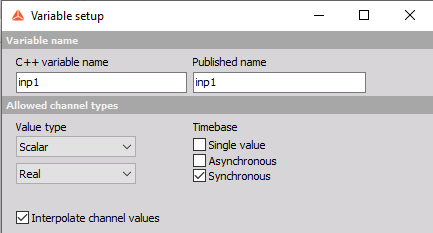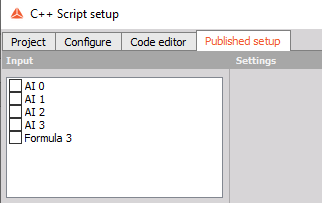The input channels for the C++ script math are predefined in the settings according to their timebase.When the Synchronous channel is selected take note that only the channels with full sample rate can be used. The channels with a divided sample rate will not be available as an input in Published setup.There are two possible workarounds, so these channels can still be used as input in the C++ script.

Solution 1:

The divided sample rate of the channel can be changed to the full sample rate through formula, where the TimeBase can be changed. For the Timebase Sync must be selected with SRDiv 1.Solution 2:

The sample rate divided synchronous channels timebase can be transformed into asynchronous with Basic statistic and then the channel can be used as an input.